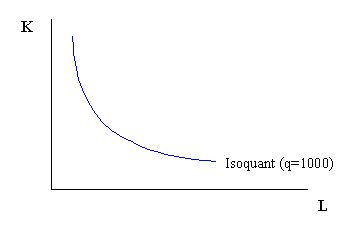# Econ 301 - Haworth

1. a. Our isoquant will tell us the different combinations of K and L that yield 1000 units of output.

Set the production function equal to 1000:                     100(KL)0.5 = 1000

Solve this for K:                                                            K = 100/L

The isoquant equation is then given as K = 100/L and should look something likeb.  Moving down the isoquant, the slope is less.  This implies that as we add single units of labor, we get an increasingly smaller reduction in the amount of capital needed (with that labor) to produce 1000 units.  Capital becomes relatively more “valuable” as we gain more labor along the isoquant.

c.  If K = 10, then we can use the isoquant equation above to determine the amount of labor hired.  According to the equation we got above, if K = 10, then L = 10 also.

d.  The average product of labor is Q/L.  Q = 1000 and L = 10, so the APL = 100.

e.  Technological change would cause the isoquant to shift inward on the graph.  The equation for the isoquant becomes K = 25/L.  Note that for every unit of L, K doesn’t have to be as large (hence a shift in the isoquant).

2. a.  To determine how many CDs to sell, John needs to produce where P = MC.  Because P is given as \$20, we set the MC equation equal to 20 and then solve for q.

20 = 0.2q + 10

q = 50

b.  John’s profits can be calculated as Price times output, less Total Costs when q = 50.

p = (P x q) – TC

p = (20 x 50) – (0.1(50)2 + 10(50) + 50)

p = 200

c. and d.  If John’s Dad charges him a flat fee or deducts half his profits, then John’s output decision doesn’t change.  In the case of the first flat fee, total cost increases by 100 (to TC = 0.1q2 + 10q + 150), but marginal costs are unchanged.  Because output is determined by where P = MC, these fees don’t affect output.  Therefore, John continues to produce 50 units.  However, because total costs are affected, John’s profits are reduced by these fees.  In both cases, his profits are reduced by half.

e.  If John’s Dad charges John a \$2 per CD sold fee, then there is a change in both his total cost and marginal cost.  Total cost increases by 2q (to TC = 0.1q2 + 12q + 50) and marginal cost increases by the amount of the \$2 fee (to MC = 0.2q + 12).  This changes output to q = 40.  As a result, profits decrease from \$200 above, to \$110 now.  His Dad collects \$80 in “fee revenue” as well.

3. a.  If the market is in long run equilibrium, then long run AC = long run MC because this is where the firms are producing when they’re at minimum AC (i.e. long run equilibrium).  Output is determined by setting the AC and MC equal to one another and then solving for q.

AC = MC

0.01q – 1 + 100/q = 0.02q – 1

q = 100

The long run AC and MC are equal, so we can plug q = 100 into either equation and solve.  Doing so, we find that AC = MC = 1.

b-d.  The long run price of gas is \$1.  This is because the price occurs where AC = MC.  Using the market demand equation, we can plug \$1 for P and solve for QD.  Doing so, we find that QD = 2,000,000.  Because each gas station produces 100 units (which we got from a, above), it will take 20,000 gas stations to supply this market.